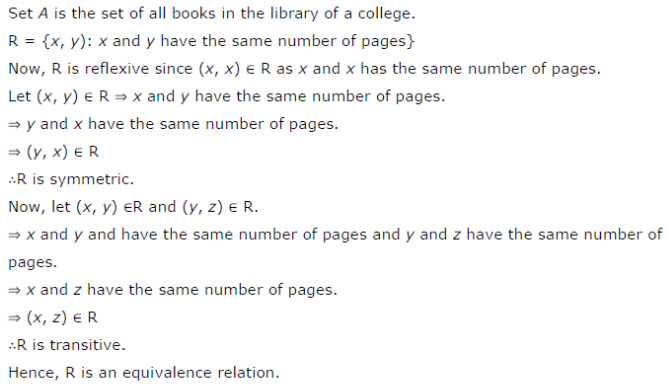# Show that the relation R in the set A of all the books in a library of a college, given by R = {(x, y):

Show that the relation R in the set A of all the books in a library of a college, given by R = {(x, y): x and y have same number of pages} is an equivalence relation.